# LIC HFL Assistant Manager Reasoning Ability Questions – (Day-01)

Dear Aspirants, Our IBPS Guide team is providing new series of Reasoning Questions for LIC HFL Assistant Manager 2019 so the aspirants can practice it on a daily basis. These questions are framed by our skilled experts after understanding your needs thoroughly. Aspirants can practice these new series questions daily to familiarize with the exact exam pattern and make your preparation effective.

Check here for LIC HFL Mock Test 2019

Check here for IBPS RRB PO Mains Mock Test 2019

Check here for IBPS RRB Clerk Mains Mock Test 2019

### Click Here to Subscribe Crack High Level Puzzles & Seating Arrangement Questions PDF 2019 Plan

[WpProQuiz 6882]

Direction (1-3): In the following question, a given question is followed by information in three statements. You have to decide the data in which statement(s) is sufficient to answer the question and mark your answer accordingly.

1) Six students P, Q, R, S, T and V study in a class. Each of them has test on different dates of November – 1st, 5th, 6th, 7th, 8th and 10th but not necessarily in the same order. Whose test is on 6th November and who is the shortest?

I) The one, whose test is on 8th November is taller than only one person. P is the tallest among all and has test on 10th November. Q is taller than those, whose tests are on 7th and 1st November.

II) The difference in the test dates of Q and T is 2. R’s test is on odd date. S is taller than Q and V.

III) T is not the shortest. Q’s test is just before S’s test. S’s test is not on any prime date.

a) All the statements are required

b) Both I and II or I and III are sufficient.

c) Only II is sufficient.

d) Only II and III are sufficient.

e) Insufficient data.

2) On which day of the week is Shefali definitely travelling?

I) Anamika correctly remembers that Shefali is travelling after Wednesday but before Sunday.

II) Mehar correctly remembers that Shefali is travelling before Saturday but after Tuesday.

III) Tessy is sure that Shefali does not travel on Fridays.

a) If the data in statements I alone is sufficient to answer the question while the data in statement II along is not sufficient to answer the question.

b) If the data in statement II alone is sufficient to answer the question while the data in statement I alone is not sufficient to answer the question.

c) If the data in statement III alone is sufficient to answer the question while the data in statement I alone is not sufficient to answer the question.

d) If the data in all the statements I, II and III together is necessary to answer the question.

e) If the data in all the statements I, II and III together to the answer due question is not sufficient.

3) On which time the Yoga is scheduled if it starts at whole number timing?

Statements:

I) It is before 02:00 PM but after 06:00 AM.

II) It is not at 10:00 AM.

III) 08:00 AM is scheduled for Breakfast and yoga is done before breakfast.

a) All the statements are required

b) Only II is sufficient

c) Only II or III and I are sufficient

d) Both I and III are sufficient

e) Question cannot be answered even with the information in all three statements

Directions (4-7): Direction: Study the following information carefully and answer the given questions.

A word and number arrangement device when given an input line of words and numbers rearranges them following a particular pattern in each step. The following is an illustration of input and rearrangement.

Input: Mike Care Wire File Yale

Step I: 4795 4986 4994 4693 4682

Step II: 4682 4693 4795 4986 4994

Step III: 6FB 7FC 9GE 10IF 8ID

Step IV: 03 09 25 07 05

Step V: X V T R B

And Step V is the last step of the above input, as the desired arrangement is obtained.

As per the logic followed in the above steps, find out in each of the following questions the appropriate step for the given input.

Input: Harp Evap Pulp Drop Atop

4) Which of the given alternatives is the last but one?

a) 09 25 01 03 05

b) 25 01 05 03 09

c) 09 05 01 25 03

d) 09 25 03 05 01

e) 25 05 03 01 09

5) What is the sum of second number from the left side and second number from the right side in the 2nd step?

a) 5129

b) 5249

c) 5419

d) 5149

e) 5229

6) Which number is obtained if we subtract second digit of second number from the left side and third digit of third number from the right in the first step?

a) 0

b) 4

c) 3

d) 1

e) 2

7) Which of the following is the third element from the left hand side in third step?

a) 7CE

b) 4FB

c) 6HD

d) 3IA

e) 10CH

Directions (8-10): Study the following information carefully and answer the given questions.

M @ N means N is the mother of M.

M # N means N is the father of M.

M % N means N is the sister of M.

M * N means N is the brother of M.

8) Which of the following expression indicates ‘K is not grandaunt of P’?

a) P @ M # N % K

b) P # M # N % K

c) P @ M @ N % K

d) P @ M % N % K

e) P # M @ N % K

9) If F # T @ K % L @ O, then how are O and F related?

a) O is F’s aunt.

b) O is F’s great-grandmother.

c) O is F’s grandmother.

d) O is F’s mother.

e) O is F’s grandaunt.

10) Which of the following expression indicates ‘T is uncle of P’?

a) P # Q @ R * T

b) P * Q # R % T

c) P * Q @ R * T

d) P # Q % R % T

e) P @ Q @ R * T

Direction (1-5) :

From statement II:

The difference in the test dates of Q and T is 2. R’s test is on odd date. S is taller than Q and V.

From the given information,

The test dates of Q and T are 5th and 7th November but not necessarily in the same order or 6th and 8th November but not necessarily in the same order. R’s test is on odd date i.e. 1st, 5th or 7th November.

So, statement II alone is not sufficient.

On combining I and II,

From the given information,

We get three different possibilities,

(i) P(10th) > S() > Q(6th) > _ (1st) > T(8th) > _ (7th)

(ii) P(10th) > S() > Q(6th) > _ (7th) > T(8th) > _ (1st)

(iii) P(10th) > S(6th) > Q(5th) > T(7th) > V(8th) > R(1st)

So, we cannot determine exactly that whose test is on 6th November and who is the shortest.

On combining I and III,

From the given information,

We get two different possibilities,

(i) P(10th) > _ > Q() > _(1st/7th) > _ (8th) > _ (7th/1st)

(ii) P(10th) > Q()> _ > _ > _(8th) > _

So, we cannot determine exactly that whose test is on 6th November and who is the shortest.

On combining II and III,

From the given information,

_ > _ > _ > _ > _ >_(×T)

So, we cannot determine exactly that whose test is on 6th November and who is the shortest.

Combining all statements together we get:

P(10th) > S(6th) > Q(5th) > T(7th) > V(8th) > R(1st)

So, S has test on 6th November and R is the shortest.

Hence, the data in statement I, II and III together are required to answer the question.

Statement I:

Shefali may be travelling on Thursday, Friday or Saturday. Hence, Statement I alone is not sufficient to answer the question.

Statement II:

Shefali may be travelling on Wednesday, Thursday or Friday. Hence, statement II alone is not sufficient to answer the question.

Statement III:

Shefali may be travelling on any day except Friday.

Statement I & II:

Shefali can be travelling on Thursday or Friday.

Statement I & III:

Shefali can be travelling on Thursday or Saturday.

Statement II and III

Shefali may be travelling on Wednesday or Thursday

Statement I, II & III:

Shefali is travelling on Thursday.

Hence, the data in all the statements I, II and III together is sufficient to answer the question.

According to given information:

Statement I. It is before 02:00 PM but after 06:00 AM.

Means Yoga is scheduled between 06:00 AM and 02:00 PM.

Statement II. It is not at 10:00 AM.

Means yoga is not scheduled at 10:00 AM

Statement III. 08:00 AM is scheduled for Breakfast and yoga is done before breakfast.

Means yoga is done before 08:00 AM

On combining statement I and II:

Statement I. It is before 02:00 PM but after 06:00 AM.

Statement II. It is not at 10:00 AM.

Here, we cannot conclude on which time the Yoga is scheduled.

On combining statement I and III:

Statement I. It is before 02:00 PM but after 06:00 AM.

Statement III. 08:00 AM is scheduled for Breakfast and yoga is done before breakfast.

We conclude that yoga is scheduled at 07:00 AM.

On combining statement II and III:

Statement II. It is not at 10:00 AM.

Statement III. 08:00 AM is scheduled for Breakfast and yoga is done before breakfast.

Here, we cannot conclude on which time the Yoga is scheduled.

Hence, both I and III are sufficient to answer this question.

Direction 4-7:

Step 1: a) Write each word in the reverse order.

b) Take the reverse alphabetical positional values of each letter.

c) If the positional value is two digit number, then take the sum of that number.

For E.g. 1) Mike → ekiM → 22 16 18 14 → 4795

Step 2: Arrange all the numbers in the ascending order.

Step 3: a) Addition of first and last number in each value.

b) The 2nd and 4th digits in the each number represents the alphabets of their respective positional values.

For E.g. 4682 → (4 + 2) = 6 → 6FB

Step 4: a) If the positional value of the last letter of the word is an even number, then add +1 to that number.

b) If the positional value of the last letter of the word is an odd number, then write the square of that number.

Step 5: a) Here, the number gives the respective alphabet as per the alphabetical positional values, take the opposite letter of that alphabet.

For E.g. The letter which has the positional value of 3 is ‘C’. Henceforth, the letter which is directly opposite to the position of C is X.

b) Arrange all the letters in the reverse alphabetical order.

Similarly the data in the input is arranged.

Input: Harp Evap Pulp Drop Atop

Step I: 2981 2854 2662 2395 2378

Step II: 2378 2395 2662 2854 2981

Step III: 10CH 7CE 4FB 6HD 3IA

Step IV: 09 25 03 05 01

Step V: Z X V R B

Direction (8-10) :

Let us first decode the given symbols and then draw a family tree.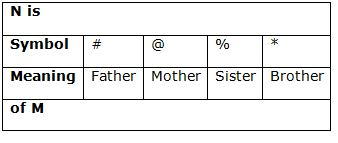Let us check each of the options;

1) P @ M # N % K

Here, this expression means,

M is the mother of P. N is the father of M. K is the sister of N.

The diagram will be as follows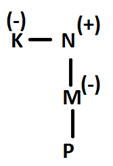Therefore, in this option K is grandaunt of P.

2) P # M # N % K

Here, this expression means,

M is the father of P. N is the father of M. K is the sister of N.

The diagram will be as follows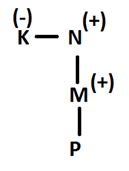Therefore, in this option K is grandaunt of P.

3) P @ M @ N % K

Here, this expression means,

M is the mother of P. N is the mother of M. K is the sister of N.

The diagram will be as follows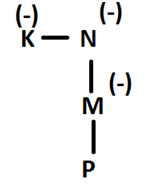Therefore, in this option K is grandaunt of P.

4) P @ M % N % K

Here, this expression means,

M is the mother of P. N is the sister of M. K is the sister of N.

The diagram will be as follows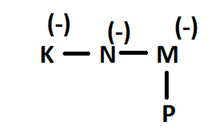Therefore, in this option K is the aunt of P.

Hence, option d is the one in which K is not grandaunt of P.

Expression F # T @ K % L @ O means that,

T is the father of F. K is the mother of T. L is the sister of K. O is the mother of L.

The diagram will be as follows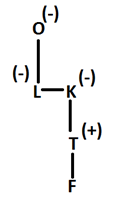Therefore, clearly, O is the great-grandmother of F.

Let us check each of the options;

1) P # Q @ R * T

Here, this expression means,

Q is the father of P. R is the mother of Q. T is the brother of R.

The diagram will be as follows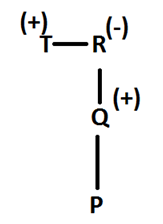Therefore, in this option T is granduncle of P.

2) P * Q # R % T

Here, this expression means,

Q is the brother of P. R is the father of Q. T is the sister of R.

Here, T is female, hence not possible.

3) P * Q @ R * T

Here, this expression means,

Q is the brother of P. R is the mother of Q. T is the brother of R.

The diagram will be as follows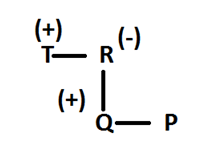Clearly, T is the uncle of P in this option.